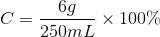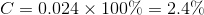# GED Science : Concentration

## Example Questions

### Example Question #1 : Chemistry

6g of sugar is dissolved in 250mL of water. What is the percent concentration of sugar in mass per unit volume?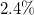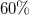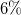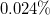Explanation:

Concentration can be measured in a variety of ways. To find the percent mass per unit volume, we must divide the mass of solute by the volume of solvent and multiply by 100%.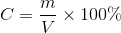We know our mass is 6g and our volume is 250mL. Use these values in the equation to find the concentration.